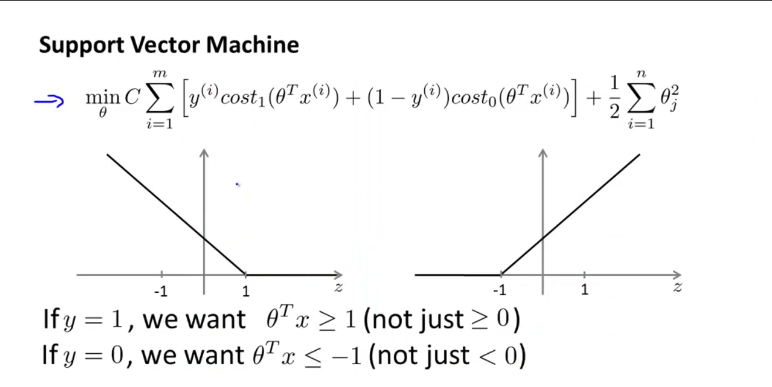## 支持向量机SVM(Support Vector Machine)

### 从逻辑回归到SVM

$J(\theta)=\frac{1}{m}\sum^m_{i=1}(-ylog(h_\theta(x))-(1-y)log(1-h_\theta(x))$

SVM hypothesis :QQ图片20160313101139.png-76.5kB

### 大间距分类器 Large Margin Intuition

#### 决策边界 Decision Boundary

margin指的是边界离样本的最近的距离，使margin最大化就是使cost function最小化的过程。因为使J变小也就是使$\frac{1}{2}||\theta||^2$变小（因为当C较大时我们会使前面那项尽可能为0，由此忽略）。但是因为$y>1$时要有$p^i*||\theta||>1$，所以要使$\theta$小的话，$p^i$就得大，也就是样本点离边界的距离要大，也就是margin要大。

#### 异常点

SVM在处理异常点上会根据C的值来给定边界。

### 核函数 Kernels

#### 不同的核函数

• 线性核函数 (没有核函数) $f^i=x^i$
• 高斯核函数$f_i=exp(1\frac{||x-l^i||^2}{2\sigma^2})$,where $l^i=x^i$
• 核函数需要满足 Mercer’s Throrem从而使用各种数值优化算法。现在其他的核函数已经不多用了，它们有
1. Polynomial kernel
2. String kernel
3. chi-square kernel等等

### 选择参数

$C=\frac{1}{\lambda}$
Large C:Lower bias,high variance.
Small C:Higher bias,low variance.
$\sigma^2$
Large:f分布更平滑，Higher bias,lower variance.
Small:较陡峭,Lower bias,Higher variance.

训练K个SVM。